Question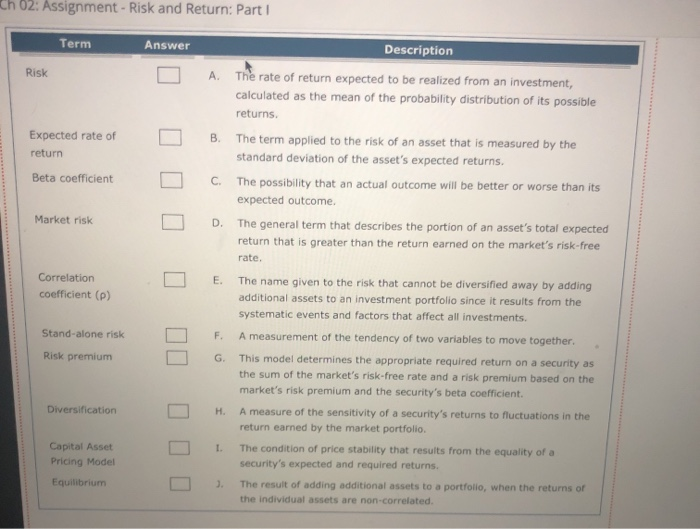Solution :

 Term Answer Risk C Expected Rate of Return A Beta Coefficient H Market Risk E Correlation Coefficient (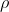) F Stand-Alone Risk B Risk Premium D Diversification J Capital Asset Pricing Model G Equilibrium I

#### Earn Coins

Coins can be redeemed for fabulous gifts.

Similar Homework Help Questions
• ### Term Description Risk A. The term applied to the risk of an asset that is measured...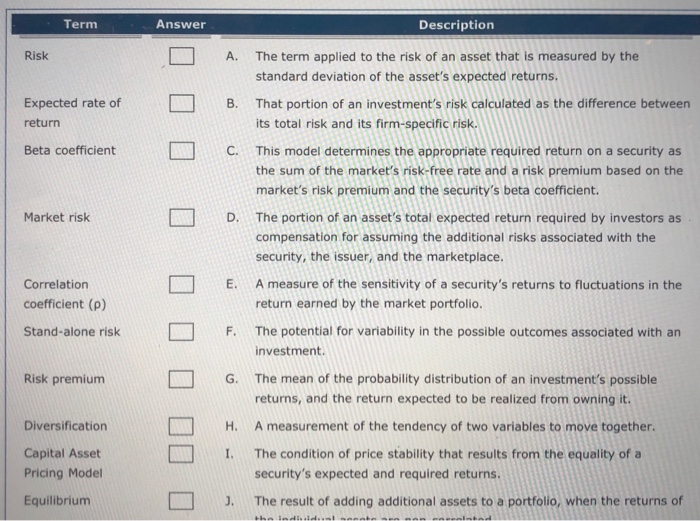Term Description Risk A. The term applied to the risk of an asset that is measured by the standard deviation of the asset's expected returns. That portion of an investment's risk calculated as the difference between its total risk and its firm-specific risk. Expected rate of return B. Beta coefficient L C . This model determines the appropriate required return on a security as the sum of the market's risk-free rate and a risk premium based on the market's risk...

• ### Term Answer Description A. The general term that describes the portion of an asset's total expected...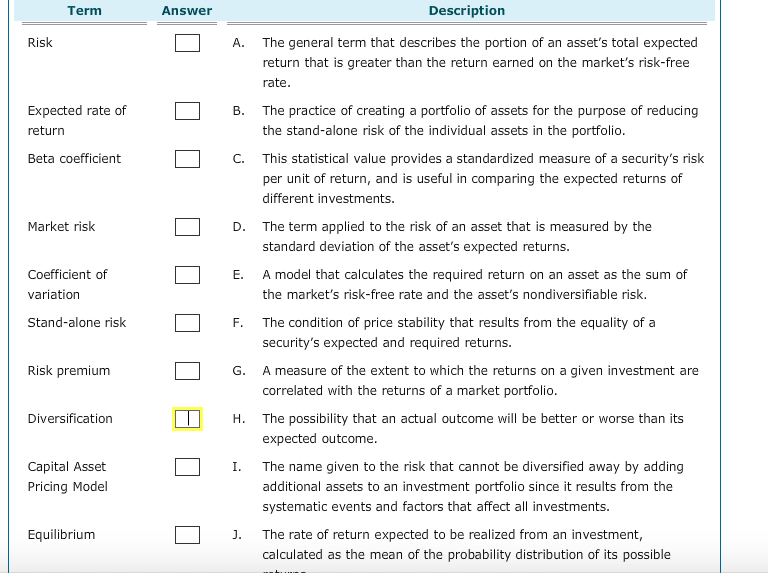Term Answer Description A. The general term that describes the portion of an asset's total expected return that is greater than the return earned on the market's risk-free rate Expected rate of return B. The practice of creating a portfolio of assets for the purpose of reducing the stand-alone risk of the individual assets in the portfolio Beta coefficient C. This statistical value provides a standardized measure of a security's risk per unit of return, and is useful in comparing...

• ### Term Answer Description Risk A. The potential for variability in the possible outcomes associated with an...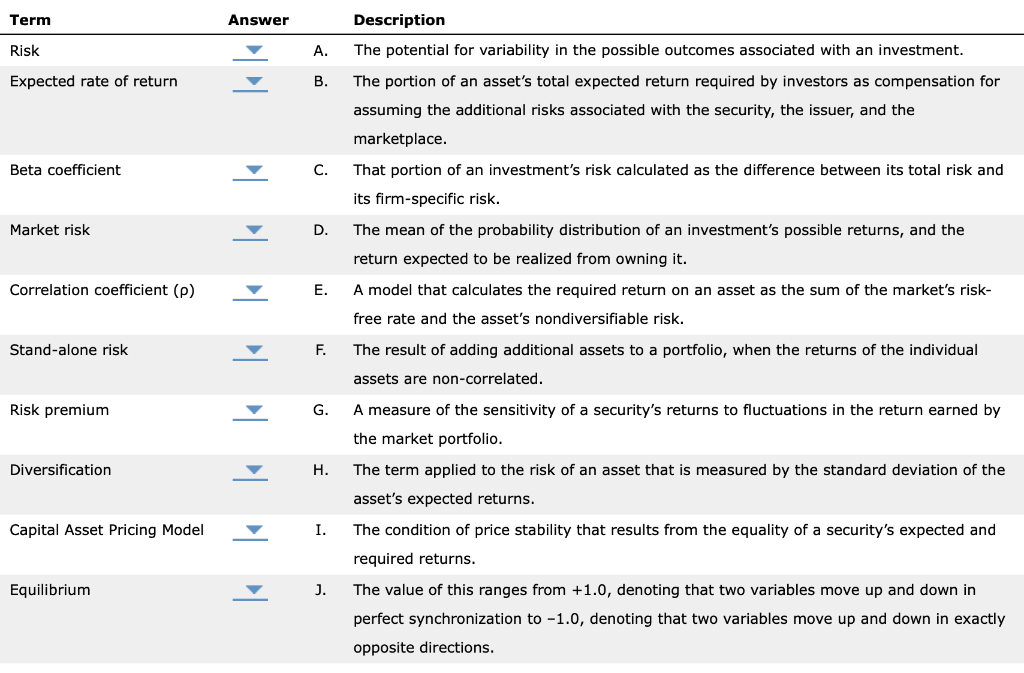Term Answer Description Risk A. The potential for variability in the possible outcomes associated with an investment. Expected rate of return The portion of an asset's total expected return required by investors as compensation for assuming the additional risks associated with the security, the issuer, and the marketplace. Beta coefficient That portion of an investment's risk calculated as the difference between its total risk and its firm-specific risk. Market risk The mean of the probability distribution of an investment's possible...

• ### Term Answer Description Risk A. The risk of an asset when it is the only asset...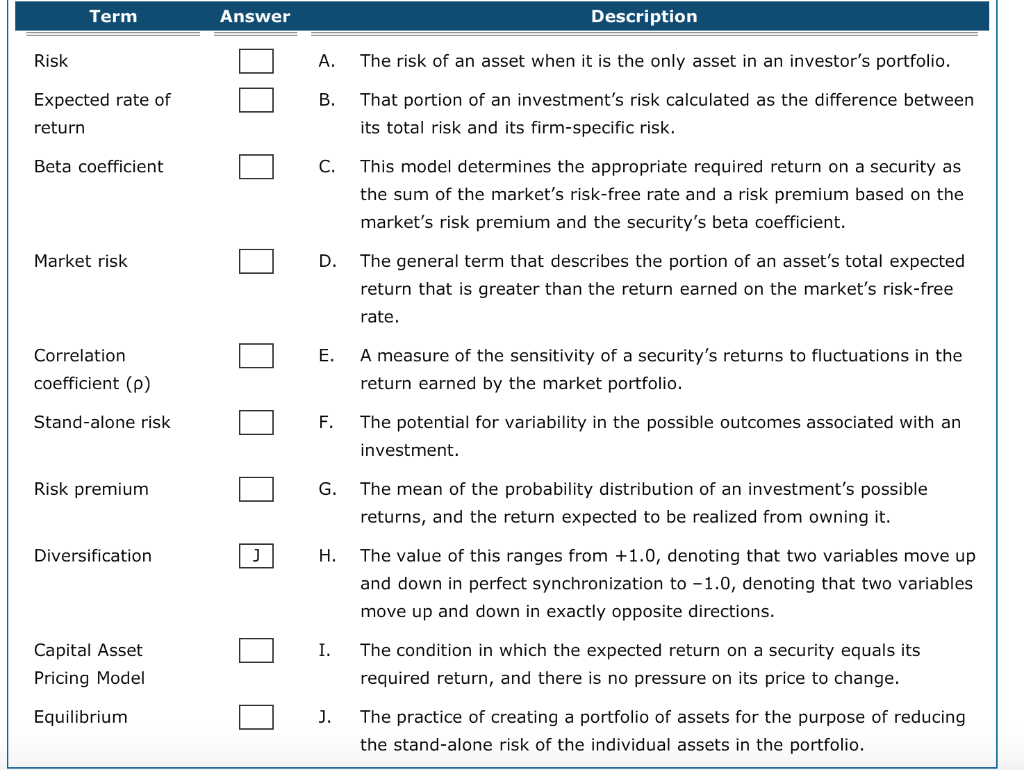Term Answer Description Risk A. The risk of an asset when it is the only asset in an investor's portfolio. Expected rate of return That portion of an investment's risk calculated as the difference between its total risk and its firm-specific risk. Beta coefficient This model determines the appropriate required return on a security as the sum of the market's risk-free rate and a risk premium based on the market's risk premium and the security's beta coefficient. Market risk The...

• ### 2. 3: Risk and Rates of Return: Risk in Portfolio Context Risk and Rates of Return:...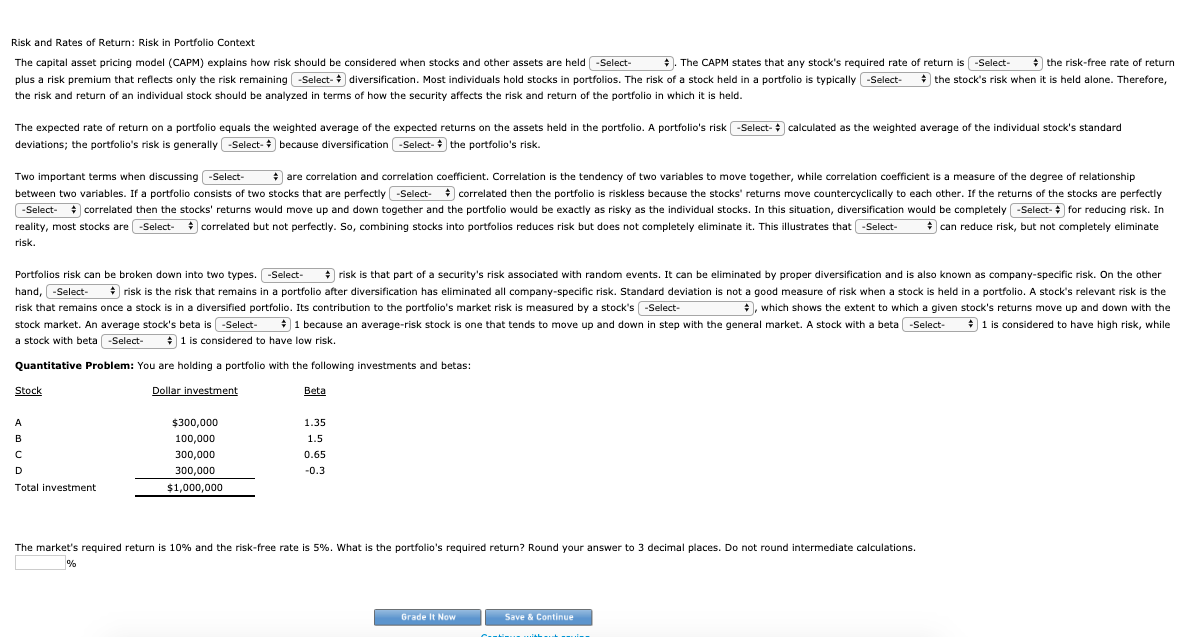2. 3: Risk and Rates of Return: Risk in Portfolio Context Risk and Rates of Return: Risk in Portfolio Context The capital asset pricing model (CAPM) explains how risk should be considered when stocks and other assets are held . The CAPM states that any stock's required rate of return is the risk-free rate of return plus a risk premium that reflects only the risk remaining diversification. Most individuals hold stocks in portfolios. The risk of a stock held in...

• ### Asset Y has a beta of 1.2. The risk-free rate of return is 6 percent, while...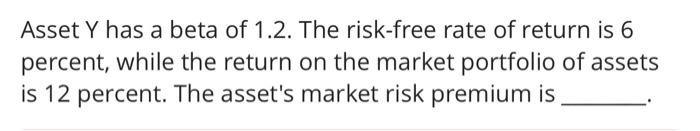Asset Y has a beta of 1.2. The risk-free rate of return is 6 percent, while the return on the market portfolio of assets is 12 percent. The asset's market risk premium is Asset Y has a beta of 1.2. The risk-free rate of return is 6 percent, while the return on the market portfolio of assets is 12 percent. The asset's market risk premium is

• ### Choose the correct answer and explain briefly 8. What is the expected return of a zero-beta...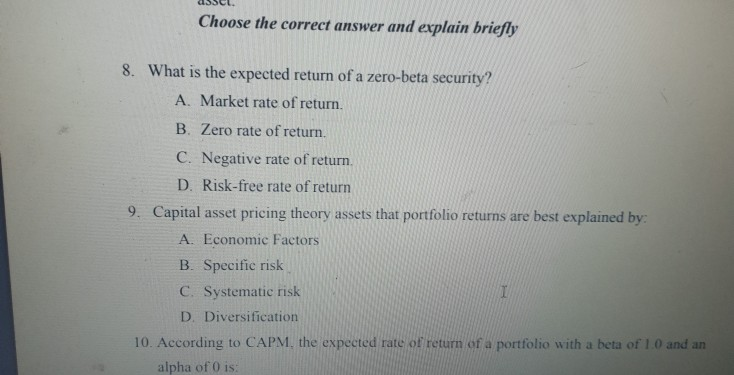Choose the correct answer and explain briefly 8. What is the expected return of a zero-beta security? A. Market rate of return. B Zero rate of return. C. Negative rate of return. D. Risk-free rate of return 9. Capital asset pricing theory assets that portfolio returns are best explained by: A. Economic Factors B. Specific risk C. Systematic risk I D. Diversification 10. According to CAPM, the expected rate of return of a portfolio with a beta of 1.0 and...

• ### The capital asset pricing model: applies to portfolios but not too individual securities. rewards investors based...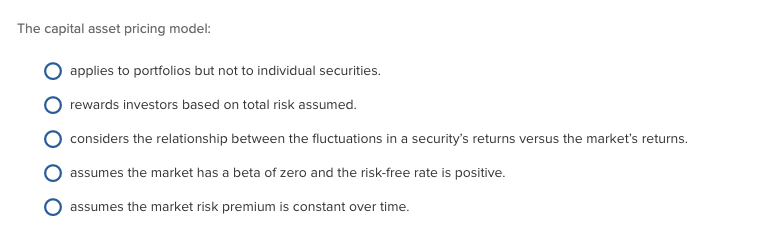The capital asset pricing model: applies to portfolios but not too individual securities. rewards investors based on total risk assumed considers the relationship between the fluctuations in a security's returns versus the market's returns assumes the market has a beta of zero and the risk-free rate is positive. assumes the market risk premium is constant over time.

• ### Risk, value of systematic risk, risk free rate of return and market risk premium

Security A = return of 15%, SD of 8%, beta of 1.2Security B = return of 12%, SD of 14%, beta of 0.90a. Which of A and B has the least total risk? The least systematic risk?b. What is the value of systematic risk for a portfolio with 75% of the funds invested in A and 25% of the funds invested in B?c. Calculate the risk free rate of return and the market risk premium (i.e., Rf and RM –...